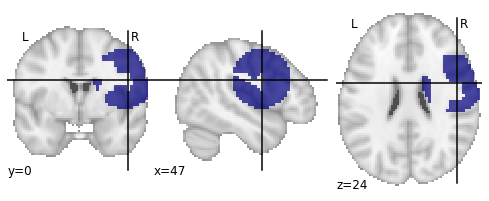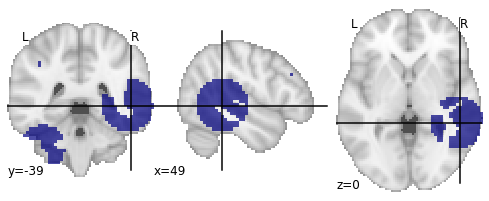# Create ROIs at random locations with fixed number of voxels

I am looking for an algorithm (best case written in Python) that I can use to ‘grow’ clusters/regions of interest in grey matter space. Is anyone aware of such an algorithm? The idea would be to give the algorithm a set of seed coordinates and a set of region sizes (i.e. number of voxels).

1.) The algorithm should then grow clusters from the seed locations until it reaches the defined size.
2.) It also should respect the space defined by the grey matter mask (that is, don’t let the clusters grow inside white matter space and don’t let them grow outside the whole brain space).
3.) Finally, clusters should be not allowed to overlap

I think you can at least hack the searchlight implementation that is in Nilearn if you’re open to having your region size based on mm distance from seed.

Basically your algorithm should calculate the affinity matrix from your grey matter mask, and for each seed return the voxels in this affinity matrix that are at a distance lower than your threshold.

Hi Thomas,

yeah, my first idea was also to use `nilearn.input_data.nifti_spheres_masker._apply_mask_and_get_affinity` for that, e.g.:

``````# one sphere of radius 10mm will be displayed
seeds = [[44,0,26]]

niimg=None,
allow_overlap=True,
)

# inverse-transform X that contains masked images with spheres

# show results
plot_roi(img)
``````I guess the radius that has to be set given a number of voxels could be inferred from using:

``````def _get_sphere_radius_from_volume(V):
return (3 * V /(4 * math.pi)) ** (1. / 3)
``````

However, using such an approach does not guarantee that you end up with a sphere that contains your defined number of voxels. If the seed is placed near white matter or near the brain surface (and the sphere is large enough) it will ‘stop there’ (see image above).

Or did you mean something different?

I guess you could change slightly _apply_mask_and_get_affinity() to use number of nearest neighbor instead of radius. l110 from current nilearn source you could define clf = neighbors.NearestNeighbors( n_neighbors=1000) instead of the radius.

For the overlapping constraint just set allow overlap=False. Then either you’re problem has a solution or the function will raise an error and you should adjust you’re desired number of voxels in each region.

Hi Thomas,

thanks for your help! Based on your suggestions I came up with the following code:

``````## Get In-Mask coordinates ####################################################

# get data indices for all '1' voxels inside mask data array

# return coordinates for those '1' voxels

affine)

## Draw random seed coordinates ###############################################

def _draw_n_random_seeds(coord_array,n_seeds,rng):
'''Draw a defined number of random coordinates from an array from voxel
coordinates'''

random_row_indices = rng.randint(coord_array.shape,size=n_seeds)
random_seeds = coord_array[random_row_indices].tolist()

return random_seeds

for idx in range(0,10):

overlap = True

while overlap:

# draw random seeds
n_seeds=n_seeds,
rng=rng)

cluster_arrays = []

for random_seed,cluster_size in zip(random_seeds,cluster_sizes):
clf = neighbors.NearestNeighbors(n_neighbors=cluster_size)
cluster_arrays.append(nearest_neighbors)

cluster_arrays_summed = sum(cluster_arrays).A

overlap = np.any((cluster_arrays_summed >= 2))

# inverse-transform cluster arrays

# show results
``````

So this creates ten binary images with random clusters of fixed sizes. The ‘overlapping’ problem is handled via the while-loop (the algorithm places random seeds somewhere in grey matter space, grows spheres of fixed sizes using `n_neighbours`, and checks if they are overlapping. If they do, it tries again). Using `n_neighbours` now guarantees that I get exactly the number of voxels that I specified for each sphere.

The only problem that is still not solved is that the NearestNeighbour-algorithm does not guarantee that each cluster is a connected component, so it can happen that the next nearest grey-matter voxel is a voxel that is not directly connected to the last voxel (creating ‘disconnected’ clusters).I guess if I also set this constraint I would have to write my own algorithm where this rule is somehow implemented (“the next nearest voxel must always be connected to the last voxel”). I will leave this thread open for now, maybe I will come up with a solution that I can post hereGreetings,

Johannes

Great that you managed !

If you add this constraint (each cluster must be a connected component) then I think you need to check nilearn/regions/rena_clustering.py especially the recursive_neighbor_agglomeration() function.

The matrix “connectivity” is what you need to enforce this local connectedness. Then the aggregation scheme has to be tailored with your constraints.

Good luck

Ha! Interesting! I’ll have a look at that thx!

Hi Thomas,

I have been trying to get an intuition on how I could use ReNA clustering for my purpose, but I am struggling with the code…I can’t find a way to define seeds here? I also don’t really understand the purpose of the connectivity matrix…

Greetings,

Johannes